Next: Classical Thermodynamics Up: Statistical Thermodynamics Previous: Laws of Thermodynamics

# Exercises

1. A box is separated by a partition that divides its volume in the ratio 3:1. The larger portion of the box contains 1000 molecules of Ne gas, and the smaller, 100 molecules of He gas. A small hole is punctured in the partition, and the system is allowed to settle down and attain an equilibrium state.
1. Find the mean number of molecules of each type on either side of the partition.
2. What is the probability of finding 1000 molecules of Ne gas in the larger partition, and 100 molecules of He gas in the smaller (in other words, the same distribution as in the initial system)?

2. Consider an isolated system consisting of a large number,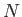, of very weakly-interacting localized particles of spin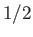. Each particle has a magnetic moment,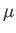, that can point either parallel or antiparallel to an applied magnetic field,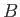. The energy,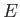, of the system is then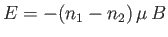, where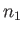is the number of spins aligned parallel to, and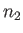the number of antiparallel spins.
1. Consider the energy range betweenand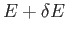, where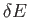is very small compared to, but is microscopically large, so that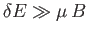. Show that the total number of states lying in this energy range is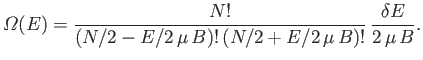2. Write down an expression for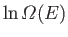. Simplify this expression using Stirling's approximation (i.e.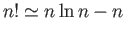) to obtain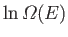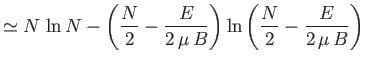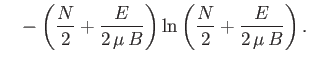3. Use the definition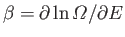(where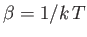) to show that the energy of the system can be written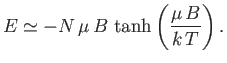4. Under what circumstances is the temperature negative?
5. Suppose that two otherwise identical spin systems with equal and opposite temperatures are brought into thermal contact. What is the final temperature of the overall system?

3. A glass bulb contains air at room temperature and at a pressure of 1 atmosphere. It is placed in a far larger chamber filled with helium gas at 1 atmosphere and at room temperature. A few months later, the experimenter happens to read in a journal that the particular glass of which the bulb is made is quite permeable to helium, but not to any other gas. Assuming that equilibrium has been reached by this time, what gas pressure will the experimenter measure inside the bulb when he/she goes back to check?

4. The heat absorbed by a mole of ideal gas in a quasi-static process in which the temperature,, changes by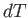, and the volume,, by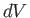, is given by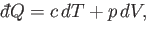where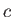is its constant molar specific heat at constant volume, and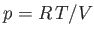is its pressure. Find an expression for the change in entropy of the gas in a quasi-static process which takes it from the initial values of temperature and volume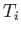and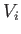, respectively, to the final values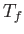and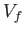, respectively. Does the answer depend on the process involved in going from the initial to the final state? What is the relationship between the temperature and the volume in an adiabatic process (i.e. a quasi-static process in which no heat is absorbed)? What is the change in entropy in an adiabatic process in which the volume changes from an initial valueto a final value?

5. A solid containsmagnetic atoms having spin. At sufficiently high temperatures, each spin is completely randomly oriented. In other words, it is equally likely to be in either one of two possible states. But at sufficiently low temperature, the interactions between the magnetic atoms causes them to exhibit ferromagnetism, with the result that their spins become oriented in the same direction. A very crude approximation suggests that the spin-dependent contribution,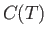, to the heat capacity of the solid has an approximate temperature dependence given by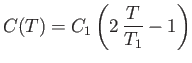for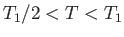, and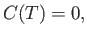otherwise. The abrupt increase in specific heat asis reduced belowis due to the onset of ferromagnetic behavior. Find two expressions for the increase in entropy as the temperature of the system is raised from a value below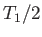to one above. By equating these two expressions, show that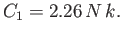Next: Classical Thermodynamics Up: Statistical Thermodynamics Previous: Laws of Thermodynamics
Richard Fitzpatrick 2016-01-25